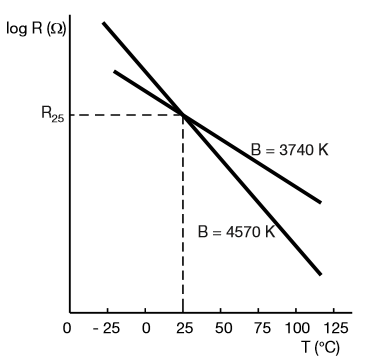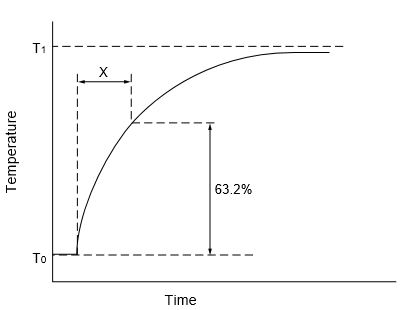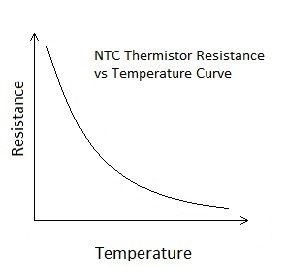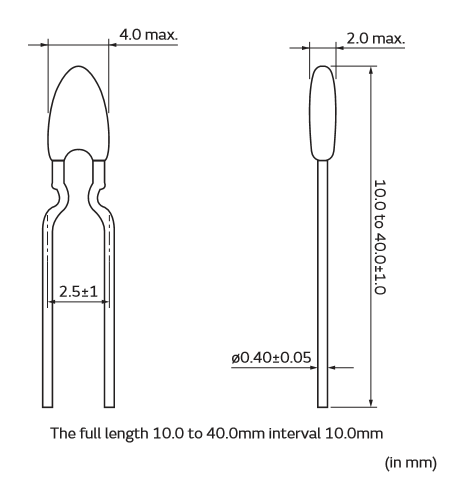# NTC Thermistor 10k

### NTC Thermistor Features

• Come with broad resistance range
• Thermistor cost is economical
• Provided with lacquer-coated thermistor disk
• Copper leads have coating of tin.
• Having lead spacing of 5.0 mm
• Component remarked with resistance and tolerance
• Good stability, durability in environment
• Provide high accuracy in resistance and B-constant
• Product is not containing lead

### Technical Specifications

• Resistance at 25 degrees C: 10K +- 1%
• B-value (material constant) = 3950+- 1%
• Dissipation factor (loss-rate of energy of a mode of oscillation) δ th = (in air)approx.7.5mW/K
• Thermal cooling time constant <= (in air) 20 seconds
• Thermistor temperature range -55 °C to 125 °C

### 1. Zero-power Resistance of Thermistor: (R)

The convenient reference point for thermistors provided by resistance is at 25 °C (substantially at room temperature).The formula by which the resistance of the thermistor is specified:

`R=R0 expB (1/T-1/T0)`

Where, R = Resistance in ambient temperature T (K)

R0 = Resistance in ambient temperature T0 (K)

B = Material constant

### 2. Material Constant: (B)

Material constant B controls the slope of the RT characteristics as shown in figure. B value vary according to the temperature and defined between two temperature 25°C and 85°C by the formula:`B25/85 = ln (R85/R25) / (1/T – 1/T0)`

B25/85 is the value used to compare and characterize different ceramics. Tolerance on this value is caused by material composition

### 3. Temperature co-efficient of Resistance: (α)

This value shows the sensitivity of a sensor according to temperature changes. It defined as:

`α = ∆ B/ T2`

The formula represents that the relative tolerance on α is equal to the relative tolerance on B-value.

### 4. Thermal Time constant

This is the time period in which the thermistor’s temperature will rapidly change 63.2% of its temperature (T0) difference from ambient temperature (T1).### 5. Thermal Dissipation Constant

The amount of electric power P (mW) consumed in T1 (ambient temperature) and T2 (rises thermistor temperature), there is a formula as follows:

`P = C (T2-T1)`

Where, C is the thermal dissipation constant.

### Brief Description

Thermistor is an electronic component used to calculate the temperature.This is a type of resistor whose resistance varies with change in temperature. These NTC thermistors are made up from the combination of metal oxides which passed through sintering process which gives negative electrical resistance versus temperature (R/T) relationship to it. Due to having a large negative slope, a small change in temperature cause a huge change in electrical resistance.

Basically, there are two types of thermistor; one is NTC (Negative Temperature Coefficient) and second one is PTC (Positive Temperature Coefficient). If the thermistor is NTC type, then it decreases the resistance as increase in temperature and PTC behavior is just opposite to the NTC. A thermistor is connected with any electrical circuit to measure the temperature of the body or the substance. This thermistor’s operating temperature range is -55 °C to 125 °C, the range of the temperature is depend upon the base resistance.The graph shows the change in the resistance with respect to the temperature, the curve is for NTC type thermistors.

### Applications

• Domestic application – Fridges, freezers, cookers and deep-fat fryers etc.
• Industrial, Telecommunication application - Process control, heating and ventilation, air conditioning, fire alarms, temperature protection in battery management/charging systems, Video and audio equipment, mobile phones and camcorders etc.
• Automotive application - Inlet air-temperature control, engine temperature control, airbag electronic systems etc.
• Thermistor can be used for temperature compensation, temperature measurement, temperature controlling.

### 2D Model and DimensionsComponent Datasheet

## Related Post

### Join 20K+subscribers

We will never spam you.

* indicates required

Be a part of our ever growing community.